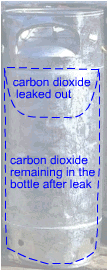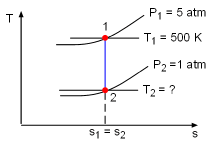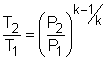Ch 6. Entropy Multimedia Engineering Thermodynamics Entropy Tds Relations EntropyChange IsentropicProcess IsentropicEfficiency EntropyBalance (1) EntropyBalance (2) ReversibleWork
 Chapter 1. Basics 2. Pure Substances 3. First Law 4. Energy Analysis 5. Second Law 6. Entropy 7. Exergy Analysis 8. Gas Power Cyc 9. Brayton Cycle 10. Rankine Cycle Appendix Basic Math Units Thermo Tables Search eBooks Dynamics Fluids Math Mechanics Statics Thermodynamics Author(s): Meirong Huang Kurt Gramoll ©Kurt GramollTHERMODYNAMICS - CASE STUDY SOLUTION A leak develops in a well-insulated bottle initially containing compressed carbon dioxide. The mass of carbon dioxide left in the bottle needs to be determined. Assumptions: No significant heat transfer occurs. Since the carbon dioxide leaks slowly, the process is assumed reversible. The carbon dioxide is modeled as an ideal gas with constant specific heats.Take the mass of carbon dioxide remaining in the bottle after leak as a systemThe Process on the T-s Diagram Molar Mass, Gas Constant for Various Common Gases Take the bottle as the system. Denote the state before leak as state 1 and the state after leak as state 2. From the assumptions made, carbon dioxide is modeled as an idea gas. With the ideal-gas equation of state, the mass remains in the bottle after leak is       m2 = P2V2/RT2 where P2 and V2 are known. If the temperature T2 is determined, the mass remaining m2 can be obtained. The gas constant R of carbon dioxide is 188.9 J(kg-K). The leaking process is adiabatic and reversible, hence it is an isentropic process. The second relation of isentropic process for ideal gases is:Assume the final temperature is 350 K and use the average value of the initial temperature and the final temperature to determine the value of k.       Tav = (500 + 350 )/2 = 425 K From the table,  k equals to 1.246 at 425 K. With all the data known, T2 can be obtained by substituting the values of T1, P1, and P2 into the above equation.       T2 = 500(1/5)0.246/1.246 = 363.9 K The calculated final temperature is close to 350 K (the assumed temperature), hence no iteration is needed. Inserting T2, P2, and V2 into the expression for m2 gives,       m2 = (101,325)(200/1,000)/((188.9)(363.9))            = 295 g